# How to use contaminante¶

This tutorial will show you how to use contaminante. If you’d like to try it yourself, you can use contaminante online, in the cloud, for free! Click here to run contaminante online using Google’s Colaboratory.

## Using contaminante on Kepler data¶

To demonstrate contaminante we’ll first use Kepler data. First we’ll need a target to try contaminante on. I’ve chosen KIC 6804648. This target was observed during the prime Kepler mission, and was flagged as a planet candidate. In fact, the target has a contaminating eclipsing binary. This binary is only obvious in some of the Kepler quarters.

Below we run the target through contaminante. Running this cell should take less than 5 minutes.

In :

import contaminante as ct
import lightkurve as lk

In :

tpfs = lk.search_targetpixelfile('KIC 6804648', quarter=[10, 11, 12]).download_all()
period = 0.700606
t0 = 131.59767
duration = 0.993/24

In :

result = ct.calculate_contamination(tpfs, period, t0, duration, plot=True, sff=False, spline_period=0.5, cbvs=True);

Modeling TPFs:   0%|          | 0/3 [00:00<?, ?it/s]/Users/ch/K2/projects/contaminante/contaminante/contaminante.py:280: RuntimeWarning: invalid value encountered in multiply
transit_pixels_err = np.zeros(tpf.flux.shape[1:]) * np.inf
/Users/ch/K2/projects/contaminante/contaminante/contaminante.py:281: RuntimeWarning: invalid value encountered in multiply
chi_ratio = np.zeros(tpf.flux.shape[1:]) * np.inf
/Users/ch/K2/projects/contaminante/contaminante/contaminante.py:405: RuntimeWarning: invalid value encountered in true_divide
aper = create_threshold_mask(thumb / err, 3) & aper
Modeling TPFs:  33%|███▎      | 1/3 [00:06<00:13,  6.64s/it]/Users/ch/K2/projects/contaminante/contaminante/contaminante.py:280: RuntimeWarning: invalid value encountered in multiply
transit_pixels_err = np.zeros(tpf.flux.shape[1:]) * np.inf
/Users/ch/K2/projects/contaminante/contaminante/contaminante.py:281: RuntimeWarning: invalid value encountered in multiply
chi_ratio = np.zeros(tpf.flux.shape[1:]) * np.inf
/Users/ch/K2/projects/contaminante/contaminante/contaminante.py:405: RuntimeWarning: invalid value encountered in true_divide
aper = create_threshold_mask(thumb / err, 3) & aper
Modeling TPFs:  67%|██████▋   | 2/3 [00:12<00:06,  6.26s/it]/Users/ch/K2/projects/contaminante/contaminante/contaminante.py:280: RuntimeWarning: invalid value encountered in multiply
transit_pixels_err = np.zeros(tpf.flux.shape[1:]) * np.inf
/Users/ch/K2/projects/contaminante/contaminante/contaminante.py:281: RuntimeWarning: invalid value encountered in multiply
chi_ratio = np.zeros(tpf.flux.shape[1:]) * np.inf
/Users/ch/K2/projects/contaminante/contaminante/contaminante.py:405: RuntimeWarning: invalid value encountered in true_divide
aper = create_threshold_mask(thumb / err, 3) & aper
Modeling TPFs: 100%|██████████| 3/3 [00:17<00:00,  5.98s/it]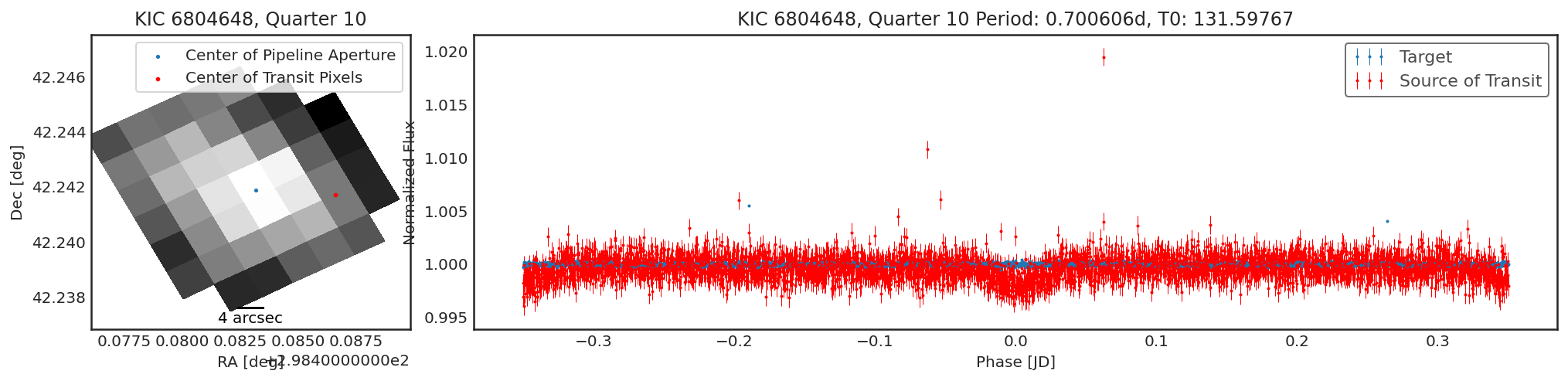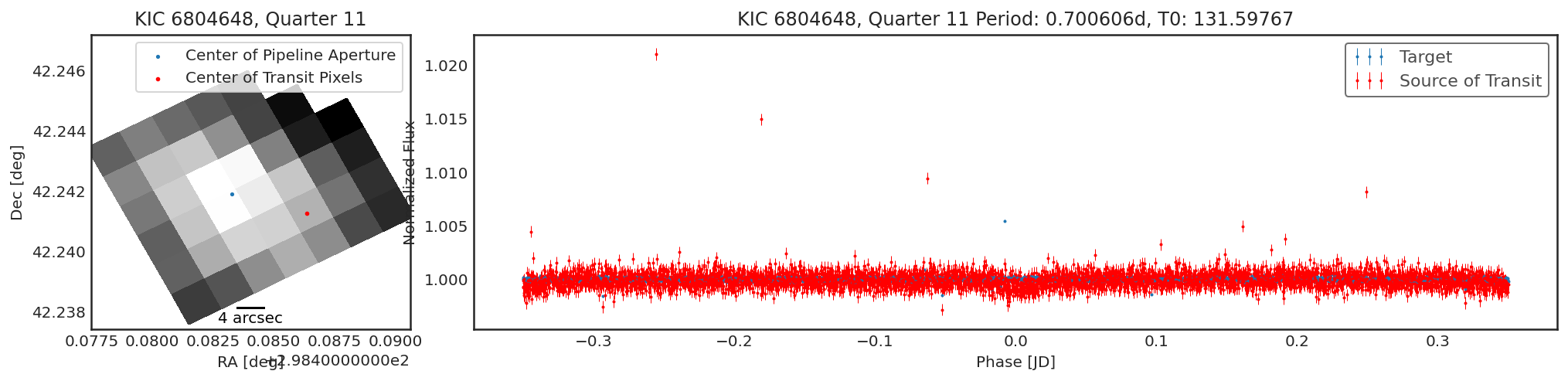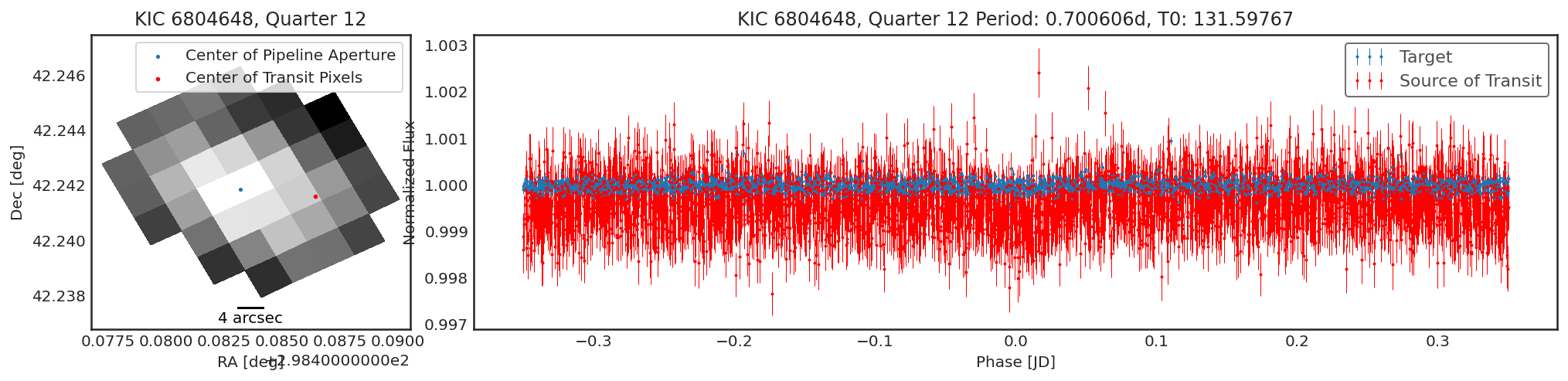Using contaminante we can see two pieces of evidence that this target is contaminated. 1. There is a significant offset between the center of the target (blue dot) in the image, and the source of the transiting signal (red dot). NOTE: The blue dot is the weighted average of the flux inside the pipeline aperture, for crowded sources this may not be accurate. 2. There is a significant difference between the target phase curve (blue phase curve) and the source of the transiting signal phase curve (red phase curve).

We name the source of the transiting signal that contaminante finds the "contaminator".

The result is a list of dictionaries contains the depth and positions of the target and the "contamintor" for each quarter, including errors. The user is encouraged to 1) look at the phase curves 2) look at the positions of the contaminator and target and 3) the transit depths of the contaminator and the target before claiming that a target is contaminated. In the above cases there is a significant difference between the contaminator and the target.

To compare, we can look at a target that is a true, confirmed planet. Below I run the parameters for Kepler-10 through contaminate.

In :

tpfs = lk.search_targetpixelfile('Kepler-10', quarter=[10, 11], cadence='long', mission='Kepler').download_all()
period = 0.837491
t0 = 2454964.57513 - 2454833
duration = 1.8076/24

result = ct.calculate_contamination(tpfs, period, t0, duration, plot=True);

Modeling TPFs:   0%|          | 0/2 [00:00<?, ?it/s]/Users/ch/K2/projects/contaminante/contaminante/contaminante.py:280: RuntimeWarning: invalid value encountered in multiply
transit_pixels_err = np.zeros(tpf.flux.shape[1:]) * np.inf
/Users/ch/K2/projects/contaminante/contaminante/contaminante.py:281: RuntimeWarning: invalid value encountered in multiply
chi_ratio = np.zeros(tpf.flux.shape[1:]) * np.inf
/Users/ch/K2/projects/contaminante/contaminante/contaminante.py:405: RuntimeWarning: invalid value encountered in true_divide
aper = create_threshold_mask(thumb / err, 3) & aper
Modeling TPFs:  50%|█████     | 1/2 [00:08<00:08,  8.10s/it]/Users/ch/K2/projects/contaminante/contaminante/contaminante.py:280: RuntimeWarning: invalid value encountered in multiply
transit_pixels_err = np.zeros(tpf.flux.shape[1:]) * np.inf
/Users/ch/K2/projects/contaminante/contaminante/contaminante.py:281: RuntimeWarning: invalid value encountered in multiply
chi_ratio = np.zeros(tpf.flux.shape[1:]) * np.inf
/Users/ch/K2/projects/contaminante/contaminante/contaminante.py:405: RuntimeWarning: invalid value encountered in true_divide
aper = create_threshold_mask(thumb / err, 3) & aper
Modeling TPFs: 100%|██████████| 2/2 [00:14<00:00,  7.40s/it]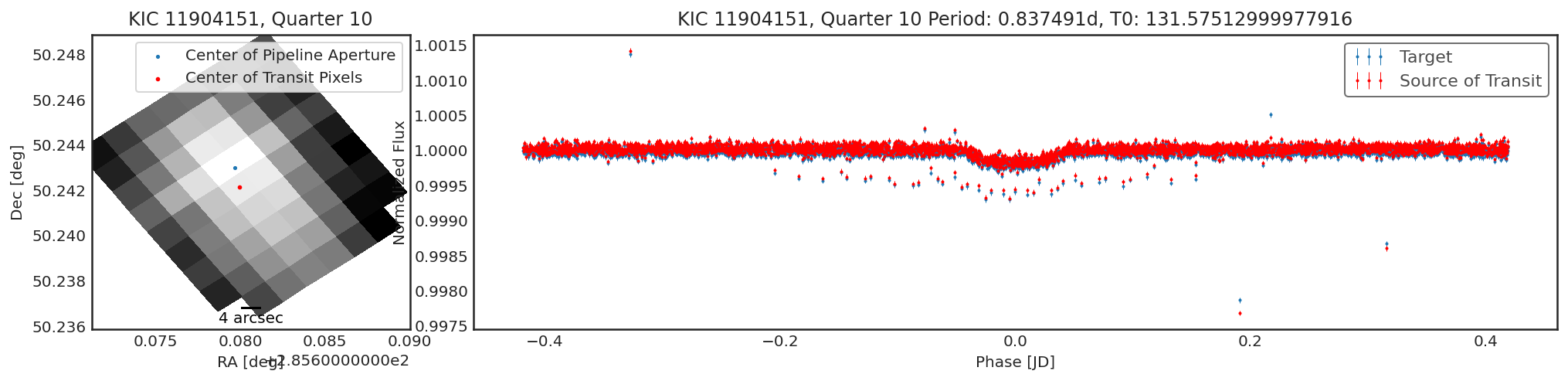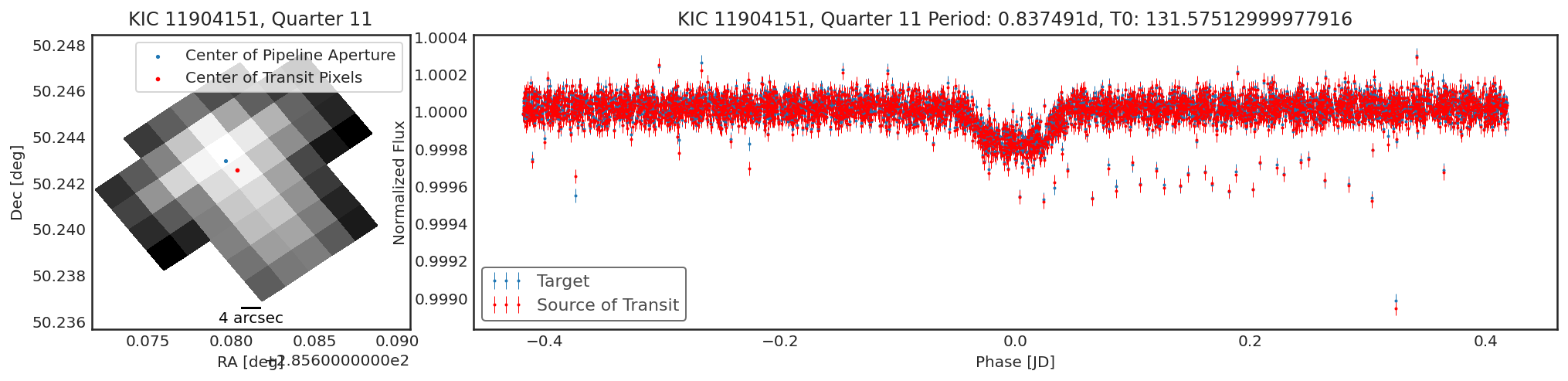Sometimes there will be no significant transiting source that was not the target, and so the contaminator and target fields will be very similar. Sometimes there will be a weak detection due to a slight difference in optimal aperture. If there is only a small change in centroid ($$<<$$ than a pixel width) and only a small change in transit depth, this would indicate there is no contaminant.

## Using contaminante on TESS Data¶

contaminante works on TESS data too. We don’t currently have a way to remove TESS background, so make sure to pass in TPFs that have already been background corrected.

In :

# TESS Data
period = 4.12688
t0 = 2458325.78297 - 2457000
duration = 0.3

result = ct.calculate_contamination(tpfs, period, t0, duration, plot=True);

/Users/ch/.pyenv/versions/3.8.0/lib/python3.8/site-packages/lightkurve/search.py:346: LightkurveWarning: Warning: 2 files available to download. Only the first file has been downloaded. Please use download_all() or specify additional criteria (e.g. quarter, campaign, or sector) to limit your search.
warnings.warn(
Modeling TPFs:   0%|          | 0/1 [00:00<?, ?it/s]/Users/ch/K2/projects/contaminante/contaminante/contaminante.py:280: RuntimeWarning: invalid value encountered in multiply
transit_pixels_err = np.zeros(tpf.flux.shape[1:]) * np.inf
/Users/ch/K2/projects/contaminante/contaminante/contaminante.py:281: RuntimeWarning: invalid value encountered in multiply
chi_ratio = np.zeros(tpf.flux.shape[1:]) * np.inf
/Users/ch/K2/projects/contaminante/contaminante/contaminante.py:600: RuntimeWarning: invalid value encountered in log10
np.log10(np.nanmedian(np.nan_to_num(tpf.flux.value), axis=0)),
Modeling TPFs: 100%|██████████| 1/1 [00:48<00:00, 48.37s/it]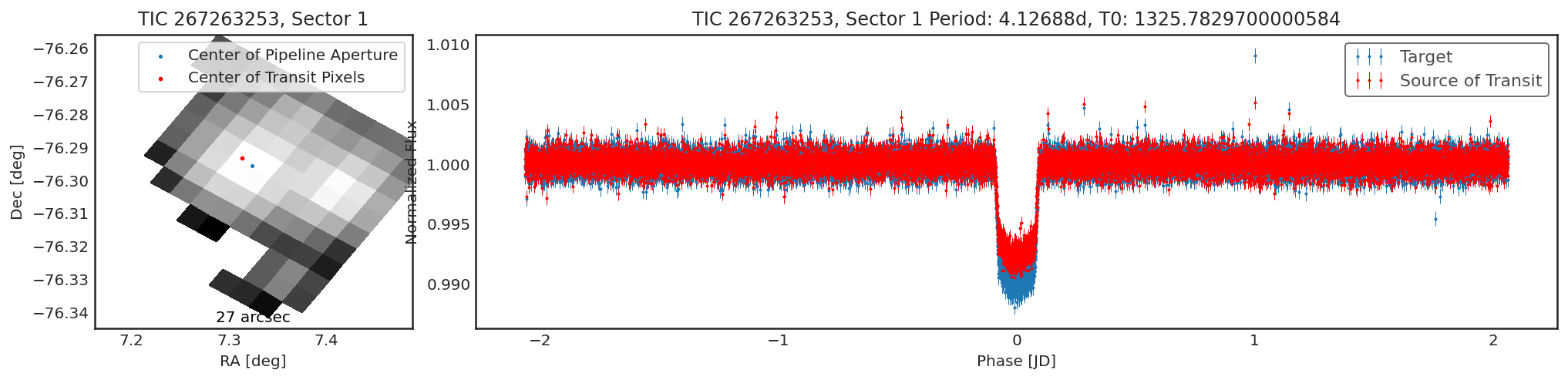In this case, it looks like there is a small offset, however the transit depth from contaminante is actually shallower. The aperture selected by contaminante is quite large, we can see this when we plot the two apertures side by side:

In :

import matplotlib.pyplot as plt
fig, ax = plt.subplots(1, 2, figsize=(8, 4))
ax.imshow(result['target_aper'], cmap='Greys')
ax.imshow(result['contaminator_aper'], cmap='Greys')
ax.set(title='Pipeline Aperture', xticks=[], yticks=[])
ax.set(title='contaminante Aperture', xticks=[], yticks=[])

Out:

[Text(0.5, 1.0, 'contaminante Aperture'), [], []]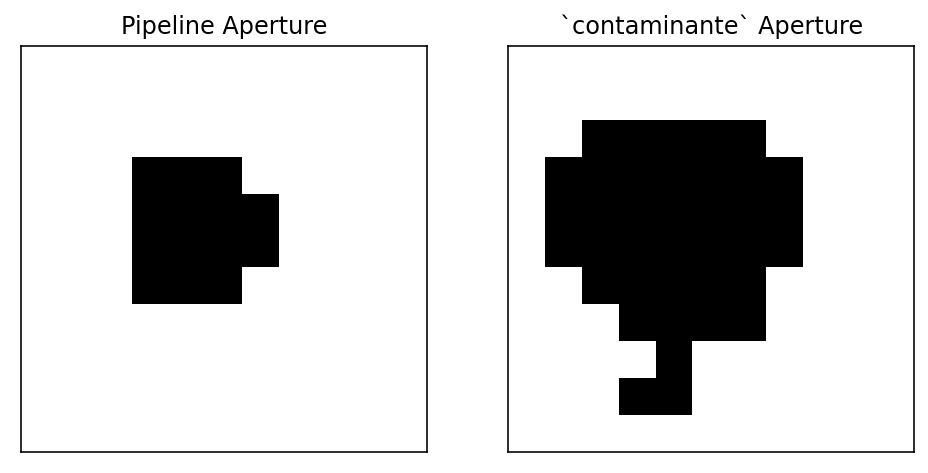This causes contaminante to produce a slightly shallower transit (because we’re including more noise and flux from objects that aren’t the target). Because the transit depth is shallower, even though there is a small offset, we shouldn’t believe this is a contaminant.

If you want to see more of where the transit is coming from, you can look at the transit depth in each pixel.

In :

plt.imshow(result['transit_depth']/result['transit_depth_err'], vmin=0, vmax=20)
plt.title("Sigificance of Transit Detection")
cbar = plt.colorbar()
cbar.set_label("Transit Depth/\n Transit Depth Err")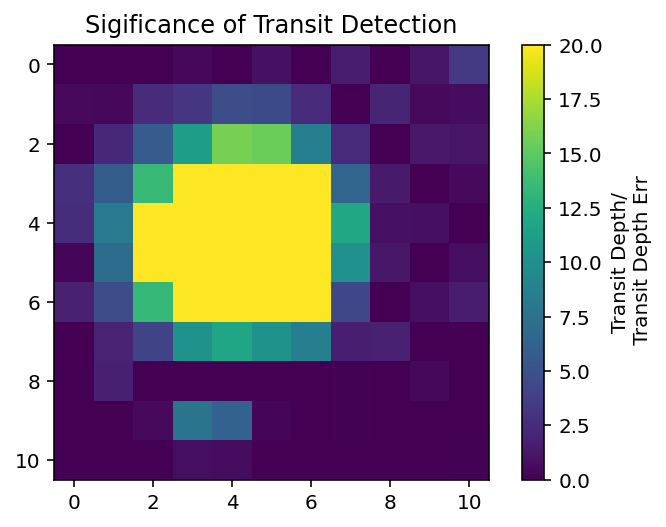## Using contaminante on K2 Data¶

contaminante works on K2 data too. The motion noise is removed using a similar approach to the Self Flat Fielding technique used in lightkurve. Because of the K2 motion the results may be a little harder to interpret. For example, below there is a slight shift in the centroid, but the light curve from that target is not different from the main target. This is likely due to the pipeline apertures for K2 being slightly too small.

Below are a couple of examples of the tool running on K2 data

In :

tpfs = lk.search_targetpixelfile("EPIC 211732801").download_all()
period = 2.1316925
t0 = 2308.407161
duration = 0.3

result = ct.calculate_contamination(tpfs, period, t0, duration, plot=True, sff=True);

Modeling TPFs:   0%|          | 0/3 [00:00<?, ?it/s]/Users/ch/K2/projects/contaminante/contaminante/contaminante.py:280: RuntimeWarning: invalid value encountered in multiply
transit_pixels_err = np.zeros(tpf.flux.shape[1:]) * np.inf
/Users/ch/K2/projects/contaminante/contaminante/contaminante.py:281: RuntimeWarning: invalid value encountered in multiply
chi_ratio = np.zeros(tpf.flux.shape[1:]) * np.inf
/Users/ch/K2/projects/contaminante/contaminante/contaminante.py:405: RuntimeWarning: invalid value encountered in true_divide
aper = create_threshold_mask(thumb / err, 3) & aper
Modeling TPFs:  33%|███▎      | 1/3 [00:18<00:37, 18.64s/it]/Users/ch/K2/projects/contaminante/contaminante/contaminante.py:280: RuntimeWarning: invalid value encountered in multiply
transit_pixels_err = np.zeros(tpf.flux.shape[1:]) * np.inf
/Users/ch/K2/projects/contaminante/contaminante/contaminante.py:281: RuntimeWarning: invalid value encountered in multiply
chi_ratio = np.zeros(tpf.flux.shape[1:]) * np.inf
/Users/ch/K2/projects/contaminante/contaminante/contaminante.py:405: RuntimeWarning: invalid value encountered in true_divide
aper = create_threshold_mask(thumb / err, 3) & aper
Modeling TPFs:  67%|██████▋   | 2/3 [00:46<00:24, 24.02s/it]/Users/ch/K2/projects/contaminante/contaminante/contaminante.py:280: RuntimeWarning: invalid value encountered in multiply
transit_pixels_err = np.zeros(tpf.flux.shape[1:]) * np.inf
/Users/ch/K2/projects/contaminante/contaminante/contaminante.py:281: RuntimeWarning: invalid value encountered in multiply
chi_ratio = np.zeros(tpf.flux.shape[1:]) * np.inf
/Users/ch/K2/projects/contaminante/contaminante/contaminante.py:405: RuntimeWarning: invalid value encountered in true_divide
aper = create_threshold_mask(thumb / err, 3) & aper
Modeling TPFs: 100%|██████████| 3/3 [00:59<00:00, 19.85s/it]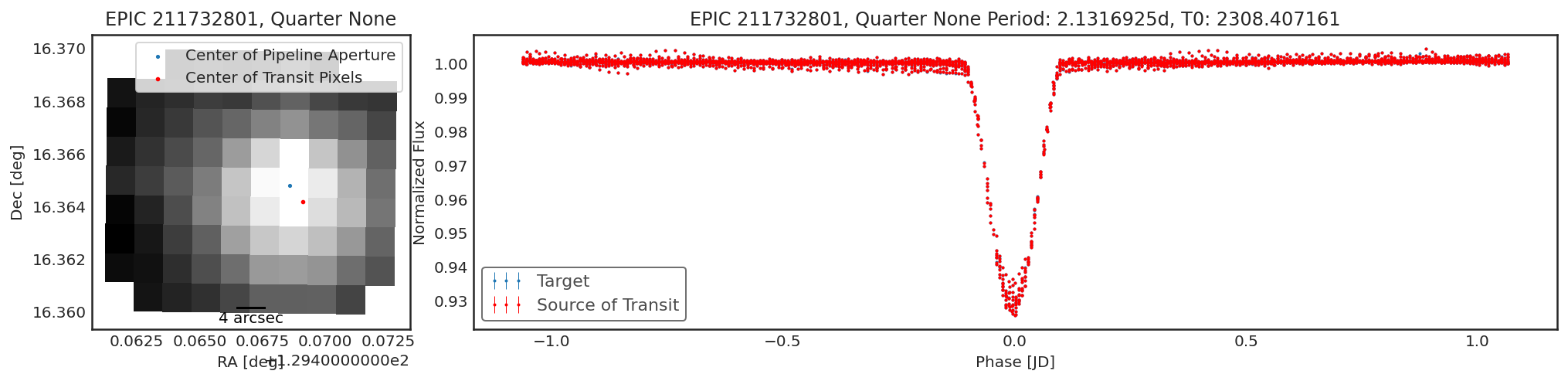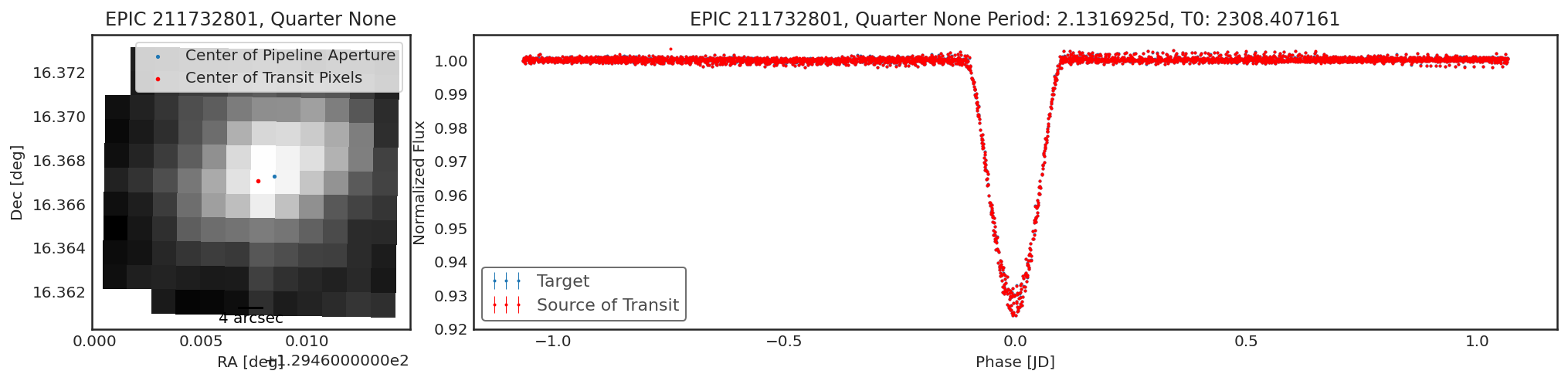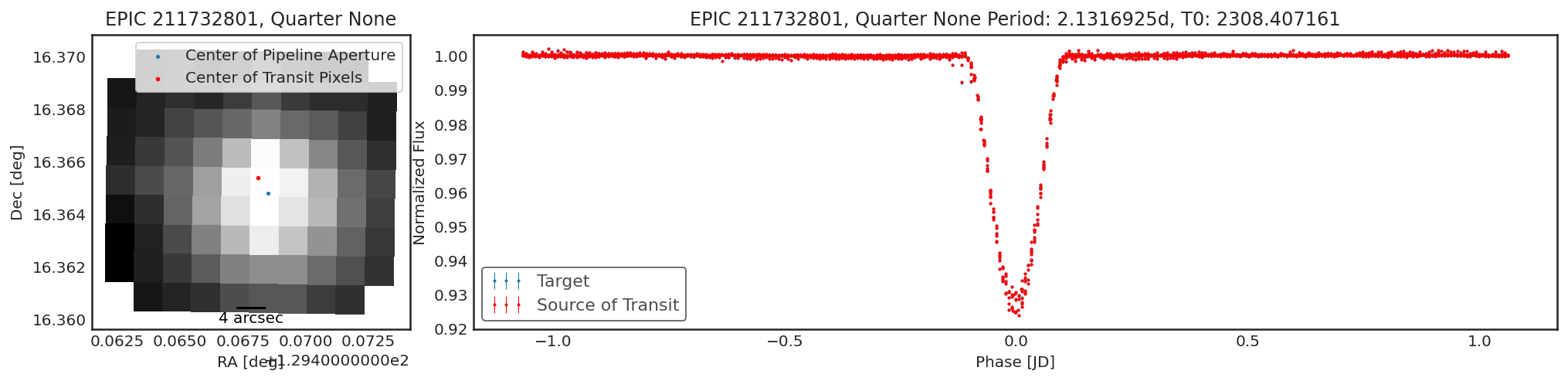In :

tpf = lk.search_targetpixelfile("EPIC 201127519").download_all()
period = 6.178369
t0 = 2457585.55131 - 2454833
duration = 0.1

# Part of the TPF is saturated from an unrelated star.
# This interferes with our result.
# We can multiply the TPF by a mask of 0s and 1s to remove this
import numpy as np

result = ct.calculate_contamination(tpf[400:] * saturation_mask, period, t0, duration, plot=True, spline_period=1.5, sff=True, sigma=10);

Warning: 25% (854/3384) of the cadences will be ignored due to the quality mask (quality_bitmask=1130799).
Modeling TPFs:   0%|          | 0/1 [00:00<?, ?it/s]/Users/ch/K2/projects/contaminante/contaminante/contaminante.py:280: RuntimeWarning: invalid value encountered in multiply
transit_pixels_err = np.zeros(tpf.flux.shape[1:]) * np.inf
/Users/ch/K2/projects/contaminante/contaminante/contaminante.py:281: RuntimeWarning: invalid value encountered in multiply
chi_ratio = np.zeros(tpf.flux.shape[1:]) * np.inf
/Users/ch/K2/projects/contaminante/contaminante/contaminante.py:405: RuntimeWarning: invalid value encountered in true_divide
aper = create_threshold_mask(thumb / err, 3) & aper
Modeling TPFs: 100%|██████████| 1/1 [00:13<00:00, 13.50s/it]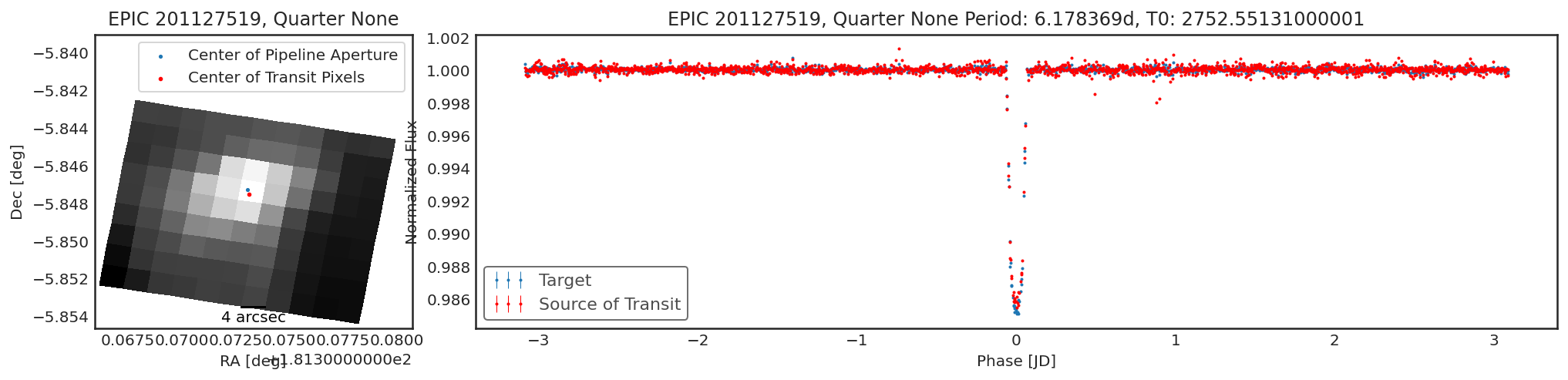contaminante does try to produce reasonable results for saturated targets, but especially for K2 this can be quite hard.

In :

tpfs = lk.search_targetpixelfile("K2-92").download_all()
period = 0.7018484
t0 = 2229.0703797
duration = 0.05
result = ct.calculate_contamination(tpfs, period, t0, duration, plot=True, sff=True, windows=20, bins=15, spline_period=1.5, cbvs=True);

Modeling TPFs:   0%|          | 0/1 [00:00<?, ?it/s]/Users/ch/K2/projects/contaminante/contaminante/contaminante.py:280: RuntimeWarning: invalid value encountered in multiply
transit_pixels_err = np.zeros(tpf.flux.shape[1:]) * np.inf
/Users/ch/K2/projects/contaminante/contaminante/contaminante.py:281: RuntimeWarning: invalid value encountered in multiply
chi_ratio = np.zeros(tpf.flux.shape[1:]) * np.inf
/Users/ch/K2/projects/contaminante/contaminante/contaminante.py:405: RuntimeWarning: invalid value encountered in true_divide
aper = create_threshold_mask(thumb / err, 3) & aper
Modeling TPFs: 100%|██████████| 1/1 [00:49<00:00, 49.90s/it]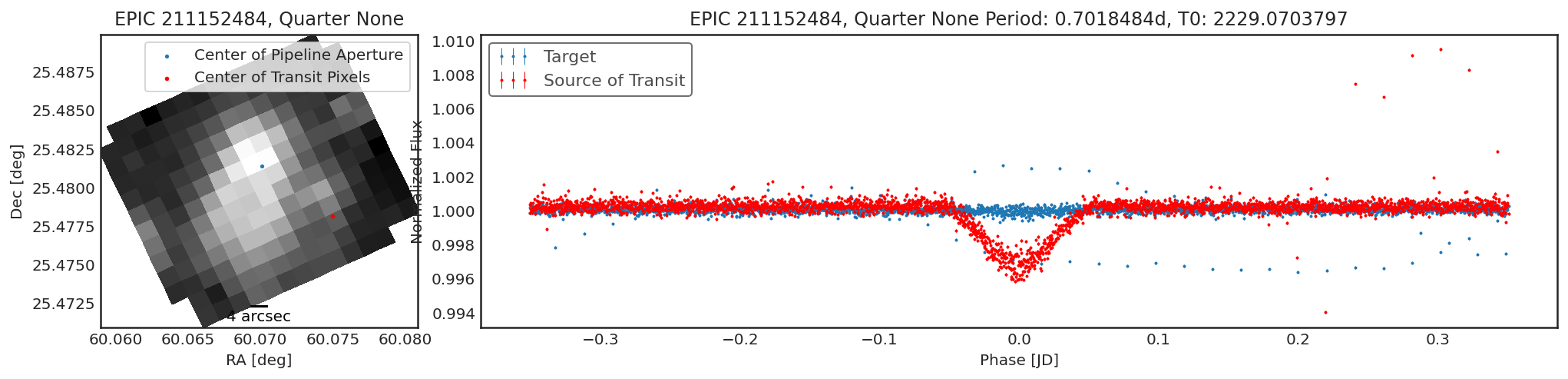In :

tpfs = lk.search_targetpixelfile("K2-78").download()
period = 2.29016
t0 = 2457065.1444 - 2454833
duration = 0.1204
result = ct.calculate_contamination(tpfs, period, t0, duration, plot=True, sff=True, windows=20, bins=5, spline_period=1., cbvs=True);

/Users/ch/.pyenv/versions/3.8.0/lib/python3.8/site-packages/lightkurve/search.py:346: LightkurveWarning: Warning: 3 files available to download. Only the first file has been downloaded. Please use download_all() or specify additional criteria (e.g. quarter, campaign, or sector) to limit your search.
warnings.warn(
Modeling TPFs:   0%|          | 0/1 [00:00<?, ?it/s]/Users/ch/K2/projects/contaminante/contaminante/contaminante.py:280: RuntimeWarning: invalid value encountered in multiply
transit_pixels_err = np.zeros(tpf.flux.shape[1:]) * np.inf
/Users/ch/K2/projects/contaminante/contaminante/contaminante.py:281: RuntimeWarning: invalid value encountered in multiply
chi_ratio = np.zeros(tpf.flux.shape[1:]) * np.inf
/Users/ch/K2/projects/contaminante/contaminante/contaminante.py:405: RuntimeWarning: invalid value encountered in true_divide
aper = create_threshold_mask(thumb / err, 3) & aper
Modeling TPFs: 100%|██████████| 1/1 [00:32<00:00, 32.01s/it]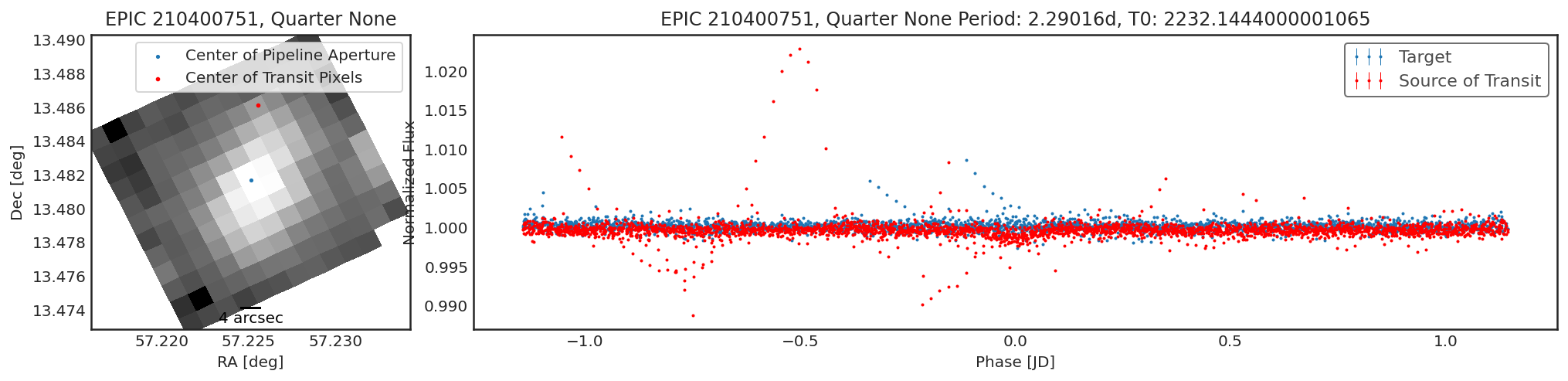We can take a look at the best contaminante aperture and compare it to the pipeline aperture:

In :

fig, ax = plt.subplots(1, 2, figsize=(10, 3))

Out:

<AxesSubplot:title={'center':'contamainante'}, xlabel='Pixel Column Number', ylabel='Pixel Row Number'>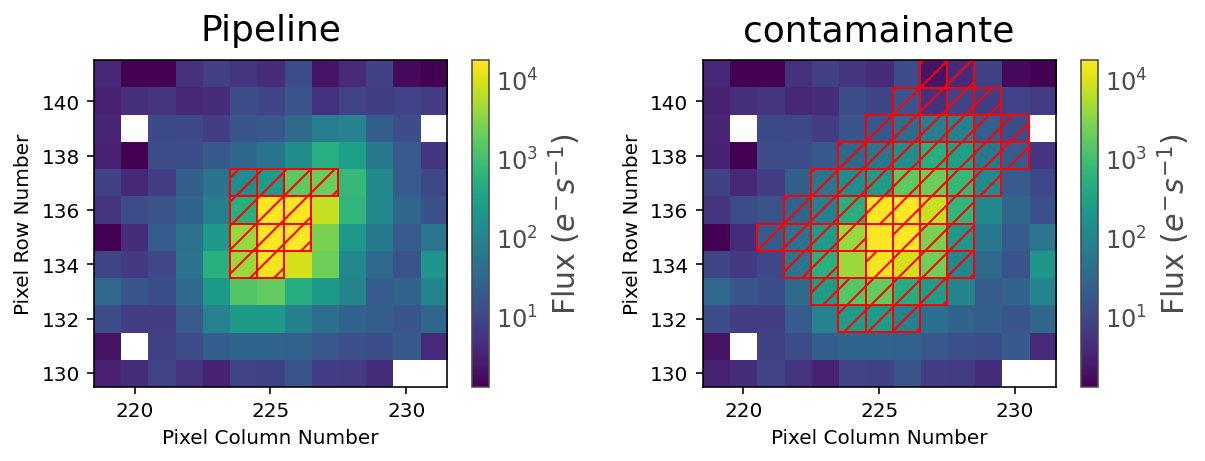2. Shallower contaminator light curves. Contaminante looks at each pixel individually to see if there is a significant transit signal. Because faint pixels can contribute a transiting signal at a lower, less significant level, some faint pixels can be missed in contaminante. In the case that the contaminator light curve is shallower than the target light curve, it is likely that some faint pixels have been missed from the optimum aperture. This does not indicate that there is any contamination.